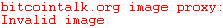## Friday, February 16, 2018

### Does neutron decay to dark matter?

Three days ago, the Quanta Magazine published a playful simple article on particle physics
Neutron Lifetime Puzzle Deepens, but No Dark Matter Seen
The neutron's lifetime is some 15 minutes but there seems to be a cool, increasingly sharp discrepancy. If you measure how many neutrons are left in a "bottle" after time $$t$$, it seems that there's one decay in 14:39 minutes. But if you measure a neutron "beam" and the protons that appear, it seems that they're being converted at the rate of one new proton per 14:48 minutes.This neutron's logo is actually from some cryptocurrency network.

So the neutrons are apparently decaying about 1% faster than the protons are born. No other decays of neutrons are known. Relativistic effects for the beam are negligible.

If this discrepancy is real, there seems to be 1% of the neutron decays that go to something else. One month ago, a paper by Bartosz Fornal, Benjamin Grinstein promoted the idea that the neutron could very well decay to new, invisible particles.

The decay would be$n\to \chi\gamma$ where $$\chi$$ is a new, dark, spin-1/2 fermion, and $$\gamma$$ is a photon. The mass of $$\chi$$ has to be just a slightly smaller than the neutron mass – a coincidance ;-) needed to avoid some sick decays in nuclear physics – and the photons $$\gamma$$ from such decays must have a completely universal, fixed energy (calculable from the $$\chi$$ mass) which is a universal constant that may a priori belong to an interval and is of order $$1\MeV$$.

In the effective Lagrangian, the decays are enabled by the quadratic and cubic terms $$\bar n \chi$$ and $$\bar n \chi \gamma$$, i.e. by a neutron-newfermion mixing; and by a cubic interaction that looks like the mixing with the extra photon (you need the photon to be represented by the whole $$F_{\mu\nu}$$ in this cubic term, not just $$A_\mu$$, by gauge invariance). So for a while, by the mixing interaction, the neutron changes to a virtual $$\chi$$, and the $$\chi$$ decays to a real neutron $$n$$ and a real photon $$\gamma$$.

The authors claim that such a model is consistent with everything we know.

One week ago, an experimental preprint claimed that they're sure that these photons of energy comparable to $$1\MeV$$ don't exist so the theory is ruled out.

Well, maybe the visible photon among the decay products should be replaced with some dark boson as well? ;-) At any rate, it's an intriguing anomaly and an equally attractive (albeit obvious) strategy to explain it.